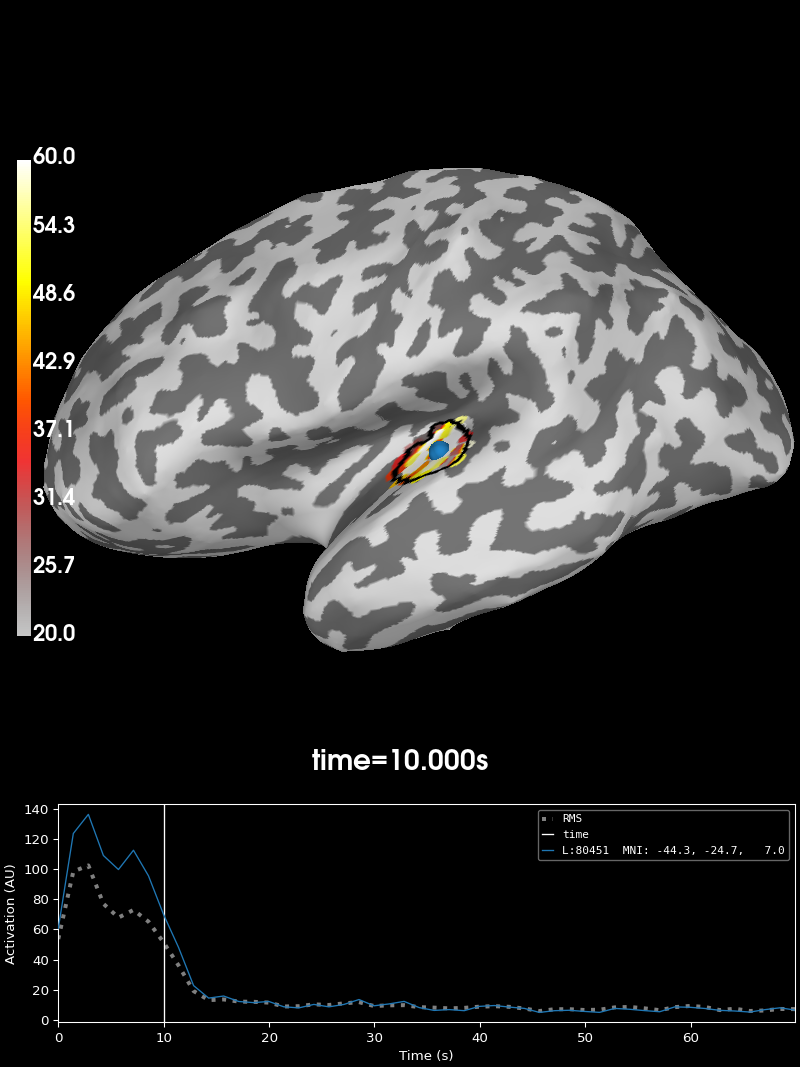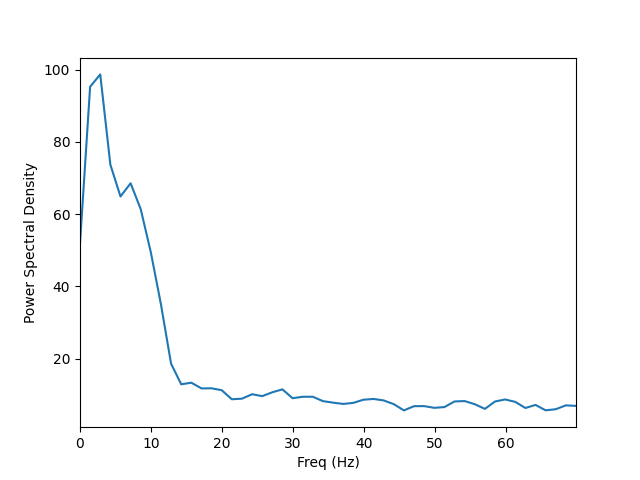# Compute Power Spectral Density of inverse solution from single epochs#

Compute PSD of dSPM inverse solution on single trial epochs restricted to a brain label. The PSD is computed using a multi-taper method with Discrete Prolate Spheroidal Sequence (DPSS) windows.

```# Author: Martin Luessi <mluessi@nmr.mgh.harvard.edu>
#
```
```import matplotlib.pyplot as plt

import mne
from mne.datasets import sample

print(__doc__)

data_path = sample.data_path()
meg_path = data_path / "MEG" / "sample"
fname_inv = meg_path / "sample_audvis-meg-oct-6-meg-inv.fif"
fname_raw = meg_path / "sample_audvis_raw.fif"
fname_event = meg_path / "sample_audvis_raw-eve.fif"
label_name = "Aud-lh"
fname_label = meg_path / "labels" / f"{label_name}.label"
subjects_dir = data_path / "subjects"

event_id, tmin, tmax = 1, -0.2, 0.5
snr = 1.0  # use smaller SNR for raw data
lambda2 = 1.0 / snr**2
method = "dSPM"  # use dSPM method (could also be MNE or sLORETA)

# Set up pick list
include = []

# pick MEG channels
picks = mne.pick_types(
raw.info, meg=True, eeg=False, stim=False, eog=True, include=include, exclude="bads"
)
epochs = mne.Epochs(
raw,
events,
event_id,
tmin,
tmax,
picks=picks,
baseline=(None, 0),
)

# define frequencies of interest
fmin, fmax = 0.0, 70.0
bandwidth = 4.0  # bandwidth of the windows in Hz
```
```Reading inverse operator decomposition from /home/circleci/mne_data/MNE-sample-data/MEG/sample/sample_audvis-meg-oct-6-meg-inv.fif...
[done]
[done]
305 x 305 full covariance (kind = 1) found.
Read a total of 4 projection items:
PCA-v1 (1 x 102) active
PCA-v2 (1 x 102) active
PCA-v3 (1 x 102) active
Average EEG reference (1 x 60) active
22494 x 22494 diagonal covariance (kind = 2) found.
22494 x 22494 diagonal covariance (kind = 6) found.
22494 x 22494 diagonal covariance (kind = 5) found.
Did not find the desired covariance matrix (kind = 3)
Computing patch statistics...
[done]
Computing patch statistics...
[done]
Read a total of 4 projection items:
PCA-v1 (1 x 102) active
PCA-v2 (1 x 102) active
PCA-v3 (1 x 102) active
Average EEG reference (1 x 60) active
Source spaces transformed to the inverse solution coordinate frame
Opening raw data file /home/circleci/mne_data/MNE-sample-data/MEG/sample/sample_audvis_raw.fif...
Read a total of 3 projection items:
PCA-v1 (1 x 102)  idle
PCA-v2 (1 x 102)  idle
PCA-v3 (1 x 102)  idle
Range : 25800 ... 192599 =     42.956 ...   320.670 secs
72 matching events found
Setting baseline interval to [-0.19979521315838786, 0.0] s
Applying baseline correction (mode: mean)
Created an SSP operator (subspace dimension = 3)
3 projection items activated
```

## Compute source space PSD in label#

..note:: By using “return_generator=True” stcs will be a generator object

instead of a list. This allows us so to iterate without having to keep everything in memory.

```n_epochs_use = 10
stcs = compute_source_psd_epochs(
epochs[:n_epochs_use],
inverse_operator,
lambda2=lambda2,
method=method,
fmin=fmin,
fmax=fmax,
bandwidth=bandwidth,
label=label,
return_generator=True,
verbose=True,
)

# compute average PSD over the first 10 epochs
psd_avg = 0.0
for i, stc in enumerate(stcs):
psd_avg += stc.data
psd_avg /= n_epochs_use
freqs = stc.times  # the frequencies are stored here
stc.data = psd_avg  # overwrite the last epoch's data with the average
```
```Considering frequencies 0 ... 70 Hz
Preparing the inverse operator for use...
Scaled noise and source covariance from nave = 1 to nave = 1
Created the regularized inverter
Created an SSP operator (subspace dimension = 3)
Created the whitener using a noise covariance matrix with rank 302 (3 small eigenvalues omitted)
Computing noise-normalization factors (dSPM)...
[done]
Picked 305 channels from the data
Computing inverse...
Eigenleads need to be weighted ...
Reducing data rank 99 -> 99
Using 2 tapers with bandwidth 4.0 Hz on at most 10 epochs

0%|          |  : 0/10 [00:00<?,       ?it/s]
10%|█         |  : 1/10 [00:00<00:00,   16.40it/s]
20%|██        |  : 2/10 [00:00<00:00,   25.76it/s]
30%|███       |  : 3/10 [00:00<00:00,   31.74it/s]
40%|████      |  : 4/10 [00:00<00:00,   35.78it/s]
50%|█████     |  : 5/10 [00:00<00:00,   39.33it/s]
70%|███████   |  : 7/10 [00:00<00:00,   44.80it/s]
90%|█████████ |  : 9/10 [00:00<00:00,   49.76it/s]
100%|██████████|  : 10/10 [00:00<00:00,   50.81it/s]
100%|██████████|  : 10/10 [00:00<00:00,   48.38it/s]
```

Visualize the 10 Hz PSD:

```brain = stc.plot(
initial_time=10.0,
hemi="lh",
views="lat",  # 10 HZ
clim=dict(kind="value", lims=(20, 40, 60)),
smoothing_steps=3,
subjects_dir=subjects_dir,
)
```Visualize the entire spectrum:

```fig, ax = plt.subplots()
ax.plot(freqs, psd_avg.mean(axis=0))
ax.set_xlabel("Freq (Hz)")
ax.set_xlim(stc.times[[0, -1]])
ax.set_ylabel("Power Spectral Density")
```Total running time of the script: (0 minutes 7.348 seconds)

Estimated memory usage: 51 MB

Gallery generated by Sphinx-Gallery